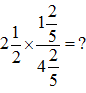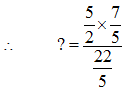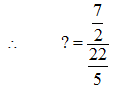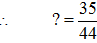Courses

# Quant Mock Test - 9

## 20 Questions MCQ Test Mock Test Series for CLAT 2020 | Quant Mock Test - 9

Description
This mock test of Quant Mock Test - 9 for CLAT helps you for every CLAT entrance exam. This contains 20 Multiple Choice Questions for CLAT Quant Mock Test - 9 (mcq) to study with solutions a complete question bank. The solved questions answers in this Quant Mock Test - 9 quiz give you a good mix of easy questions and tough questions. CLAT students definitely take this Quant Mock Test - 9 exercise for a better result in the exam. You can find other Quant Mock Test - 9 extra questions, long questions & short questions for CLAT on EduRev as well by searching above.
QUESTION: 1

Solution:
QUESTION: 2

### A man can row 10.2 km downstream in 18 min. If the speed of the stream in 3.5 km/h, how much time (in h) he would take to cover 121.5 km upstream?

Solution:

Let the speed of boat be x km/hr and speed of stream be y km/hr.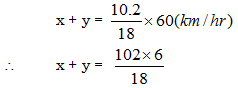∴ x + y = 34 km/hr
and y = 3.5 km/hr
∴ x = (34 – 3.5) km/hr
= 30.5 km/hr

Now for upstream
x – y = (30.5 – 3.5) km/hr
= 27 km/hr

∴ In 1 hr, distance covered is 27 km
∴ To cover 121.5 km distance, time taken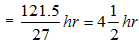∴ Option (a) is correct.

QUESTION: 3

### The respective ratio of two number is 16 : 21. If the first number is increased by 30% and the second number is decreased by 20%, what will be the respective ratio of the first and the second number?

Solution:

Let the first number be 16x
and second number be 21x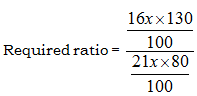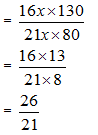∴ Option (b) is correct.

QUESTION: 4

Directions (Qs. 4 - 6): What will come in place of question mark (?) in the given number series?

Q. 29        31        37        49        69  ?

Solution:

The given series is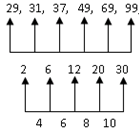∴ Option (b) is correct.

QUESTION: 5

13        13        20        37.5    83        ?

Solution:

The correct option is B.

13×0.5+6.5=13

13×0.5+6.5=13

13×1+7=20

13×1+7=20

20×1.5+7.5=37.5

20×1.5+7.5=37.5

37.5×2+8=83

37.5×2+8=83

83×2.5+8.5=216

QUESTION: 6

17        16        30        87        344     ?

Solution:
QUESTION: 7

A started a business by investing Rs. 33600. After three month B join by investing Rs. 23100. After 3 month of B’s investment. C joined them by investing Rs. 18900. If the total profit earned by them is Rs. 26450, what is C’s share of profit?

Solution:
QUESTION: 8

The sum of two numbers is equal to 27 and their product is equal to 182. What are the two numbers?

Solution:

Let the two numbers be x and y
x + y = 27         …..(i)
and      xy = 182           …..(ii)
Now (x + y)2 = (x + y)2 + 4xy
∴ (27)2 = (x – y)2 + 4(182)
∴ (x - y)2 = 729 – 728
∴ x – y = 1         ….. (iii)

Solving equations (i) and (iii) we get
x = 14 and y = 13
∴ Option (d) is correct

QUESTION: 9

Raju purchases 550 mL of milk everyday. If cost of 1 L of milk is Rs. 44, howmuch amount will be pays in 45 days?

Solution:

Cost of 1000 mL milk = Rs 44
Cost of milk for 45 days = 550 x 45 = 24750 mL.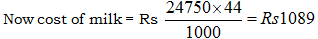∴ Option (d) is correct.

QUESTION: 10

A train 350 m long rakes 36s to cross a man running at a speed of 5 km/h in the direction opposite to that of train. What is the speed of the train?

Solution: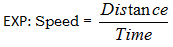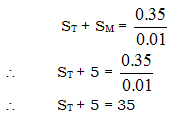∴ ST = 30 km/hr
∴ Option (a) is correct

QUESTION: 11

Directions (Q. 11 - 15): Study the table and answer the given questions.

Number of books sold by 5 stores during 5 months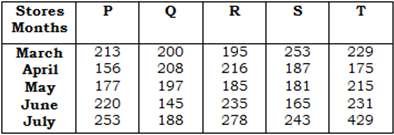Q.

Number of books sold by store P in May in approximately what percent less than the number of books sold by store T in July

Solution:

Number of book sold by store ‘P’ in May = 177
and number of book sold by store T in July = 249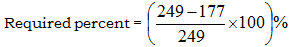=28.9% ≈ 29%
∴ Option (d) is correct

QUESTION: 12

Number of books sold by 5 stores during 5 monthsQ.

What is the respective ratio between the total number of books sold by store P in April and June together and total number of books sold by store T in May and July together?

Solution:

Total number of book sold by store ‘P’ in April and June = 156 + 220 = 376

and total number of book sold by store ‘T’ in May and July = 215 + 249 = 464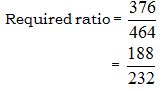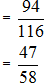∴ Option (c) is correct.

QUESTION: 13

Number of books sold by 5 stores during 5 monthsQ.

If 30% of the total number of books sold by store Q, S and T together in April were Academic books, how many non-academic books were sold by the same stores together in the same month?

Solution:

Total number of book sold by store ‘Q’, ‘S’ and ‘T’ in April = 208 + 187 + 175 = 570
Now 30% of book is academic
∴ Total number of Non-academic book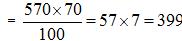∴ Option (d) is correct.

QUESTION: 14

Number of books sold by 5 stores during 5 monthsQ.

What is the average number of books sold by store R in April, June and July together?

Solution:

Average number of books sold by store R in April June and July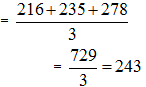∴ Option (a) is correct

QUESTION: 15

Number of books sold by 5 stores during 5 monthsQ.

What is thedifference between total number of books sold by store Q in May and July together and total number of books sold by store S in March and June together?

Solution:

Total number of books sold by store ‘Q’ in May and July = 197 + 188 = 385 and total number of books sold by store ‘S’ in March and June = 253 + 265 = 518
∴ Required difference = 518 – 385 = 133
∴ Option (d) is correct.

QUESTION: 16

The present population of village P is 2.5 times the present population of village Q. If after a year the population of village Q is 16537 and has been increased at a rate of 15%. What is the present population of village P?

Solution:

Let the present population of village Q be x
∴ Present population of village P will be 2.5x

Now according to question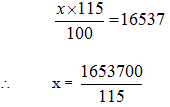= 14380
Hence present population of village P
= 2.5 x 1.4380
= 35950
∴Option (d) is correct.

QUESTION: 17

Directions (Qs. 17 - 20): What will come in place of question mark (?) in the given questions?

Q.

54.2 + 13.52 – 0.52 – 0.5636 – 0.07 = ?

Solution:

54.2 + 13.52 – 0.52 – 0.5656 – 0.07 = ?
∴ (54.2 + 13.52) – (0.52 + 0.5656 + 0.07) =?
∴ ? = 67.72 – 1.1556
∴ ? = 66.57

∴ Option (d) is correct.

QUESTION: 18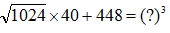Solution: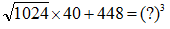∴ 32 x 40 + 448 = (?)3
∴ (?)3 = 1280 + 448
∴ (?)3 = 1728
∴  ? = 12

∴         Option (d) is correct

QUESTION: 19

255.4 + 542.3 - ? = 1014.3 – 499.4

Solution:

255.4 + 542.3 - ? = 1014.3 – 499.4
∴ ? = 255.4 + 542.3 – 1014.3 + 499.4
∴ ? = 282.8

∴        Option (d) is correct

QUESTION: 20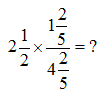Solution: